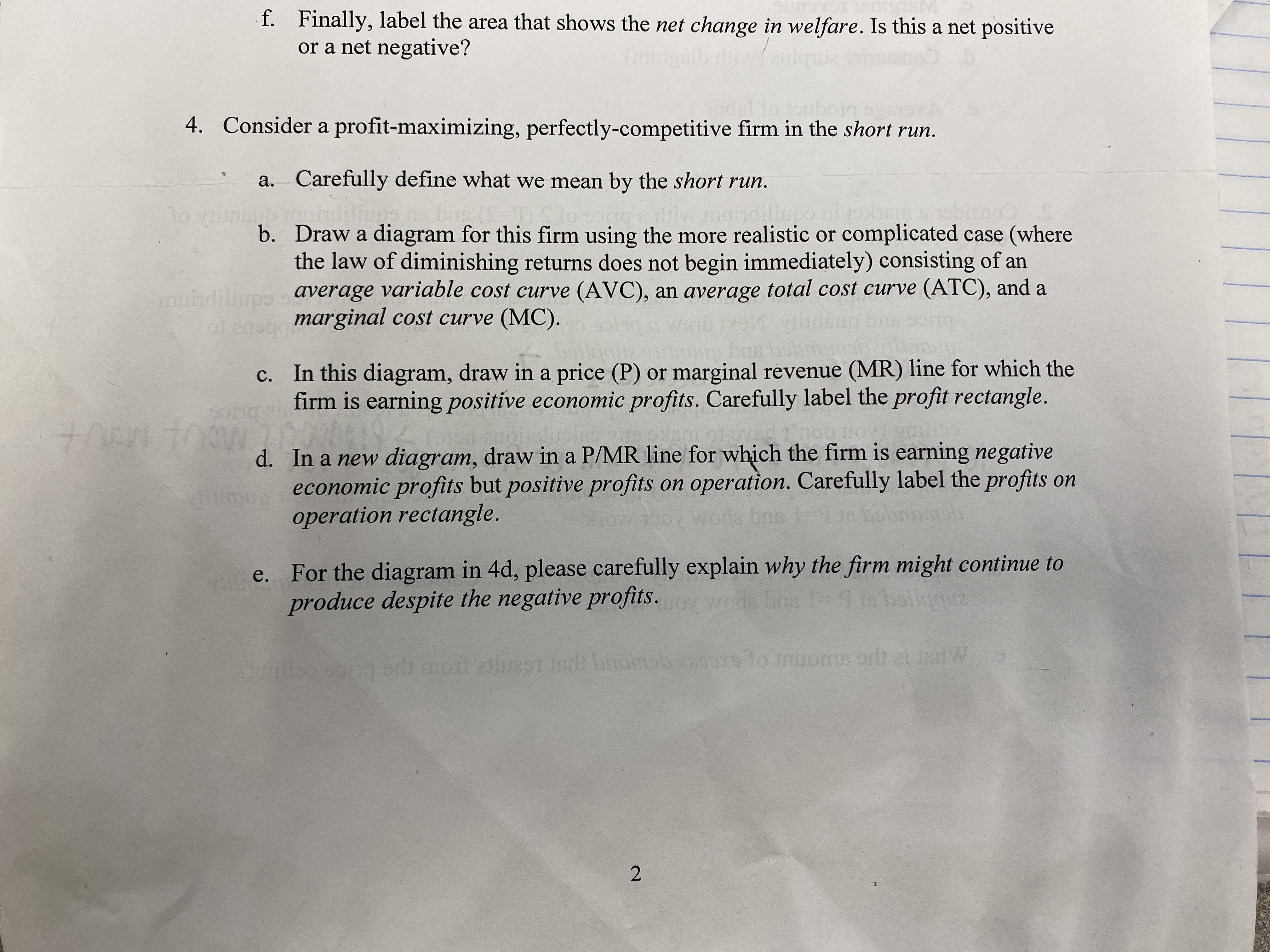# f. Finally, label the area that shows the net change in welfare. Is this a net positiveor a net negative?brognor o4. Consider a profit-maximizing, perfectly-competitive firm in the short run.a. Carefully define what we mean by the short run.bis ()dillupanohdilib. Draw a diagram for this firm using the more realistic or complicated case (wherethe law of diminishing returns does not begin immediately) consisting of anaverage variable cost curve (AVC), an average total cost curve (ATC), and amarginal cost curve (MC).muindiliupsc. In this diagram, draw in a price (P) or marginal revenue (MR) line for which thefirm is earning positive economic profits. Carefully label the profit rectangle.pisricd. In a new diagram, draw in a P/MR line for which the firm is earning negatives economic profits but positive profits on operation. Carefully label the profits onoperation rectangle.100odbase. For the diagram in 4d, please carefully explain why the firm might continue toproduce despite the negative profits.wode bns 1=-4 in boilgquzSpnilisg sdt moil alues e msb 2290x9 1o nuoms ordt at sdW

Question
87 views

Hello, I am having problems with the problems attached to this picture.help_outlineImage Transcriptionclosef. Finally, label the area that shows the net change in welfare. Is this a net positive or a net negative? brognor o 4. Consider a profit-maximizing, perfectly-competitive firm in the short run. a. Carefully define what we mean by the short run. bis () dillup ano hdili b. Draw a diagram for this firm using the more realistic or complicated case (where the law of diminishing returns does not begin immediately) consisting of an average variable cost curve (AVC), an average total cost curve (ATC), and a marginal cost curve (MC). muindiliups c. In this diagram, draw in a price (P) or marginal revenue (MR) line for which the firm is earning positive economic profits. Carefully label the profit rectangle. pisric d. In a new diagram, draw in a P/MR line for which the firm is earning negative s economic profits but positive profits on operation. Carefully label the profits on operation rectangle. 100 od bas e. For the diagram in 4d, please carefully explain why the firm might continue to produce despite the negative profits. wode bns 1= -4 in boilgquz Spnilis g sdt moil alues e msb 2290x9 1o nuoms ordt at sdW fullscreen
check_circle

Step 1

Since there are many sub part questions present in the question, only the first three sub part swill be answered her. If answer for any other sub part is needed, please repost the question by mentioning that sub part separately.

Step 2

a.

The short run is a period of time in which at least one of the factors of production of the firm is fixed or cannot be changed. Thus, the short run is the period of time which does not allows all the factors of production to become variable. When all the factors of production are variable, it means that all the variables can be changed by the firm and thus, the period is long run. There is no specific duration of time to determine whether the period is short run or long run. The difference occurs with the variability of factors of production. When all factors are variable, then the period is long run and when at least one is not variable, it is short run.

Step 3

b.

The profit maximization is the main objective of all the firms in the economy. The chances for earning higher profits are high with the imperfect markets such as the monopoly, duopoly, oligo...

### Want to see the full answer?

See Solution

#### Want to see this answer and more?

Solutions are written by subject experts who are available 24/7. Questions are typically answered within 1 hour.*

See Solution
*Response times may vary by subject and question.
Tagged in